# AP Chemistry : Compounds and Molecules

## Example Questions

← Previous 1 3 4 5 6 7 8 9 13 14

### Example Question #1 : Ap Chemistry

What is the percent by mass of bismuth in the compound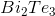?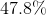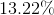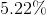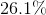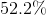Explanation:

The mass percentage of bismuth in the compound will be equal to the mass of bismuth in one mole of compound divided by the total molar mass of the compound.

Bismuth has a molar mass of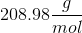. One mole of the compound would result in two moles of bismuth, a total of 417.96g.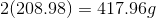Tellurium has a molar mass of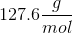. One mole of the compound would result in three moles of tellurium, a total of 382.8g.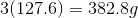Add the mass of bismuth and the mass of tellurium per mole to find the total molar mass.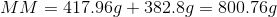Divide the mass of bismuth by the total molecular mass to find the percent by mass of bismuth in the compound.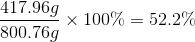### Example Question #2 : Ap Chemistry

What is the percent by mass of carbon in acetic acid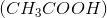?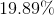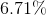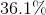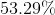Explanation:

The total molar mass of acetic acid is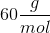.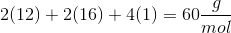Carbon contributes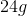total. Therefore, in a sample of 1 mole of acetic acid, there areof carbon. To find the percent by mass, we must divide the mass of carbon by the total molar mass of the compound.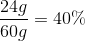### Example Question #3 : Ap Chemistry

Calculate the percent by mass of each element in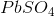.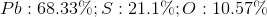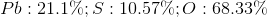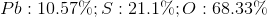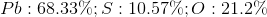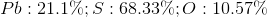Explanation:

The total molar mass of lead (II) sulfate is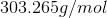. Lead contributes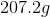, sulfur contributes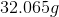, and oxygen contributes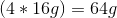.

The percent by mass of each element in the compound is found by dividing the mass contribution of that element by the total molar mass of the compound.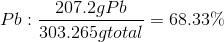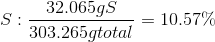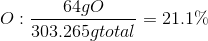### Example Question #4 : Ap Chemistry

What percent by mass of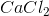is chlorine?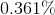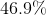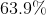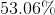Explanation:

The molar mass of calcium chloride is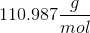. Chlorine has two moles per one mole of. Therefore, chloride contributes 70.9g to the total molecular mass.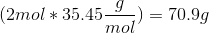To find the percent by mass of chlorine in calcium chloride, divide the contribution of chlorine by the total molar mass.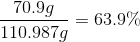### Example Question #5 : Ap Chemistry

Calculate the percent by mass of each element in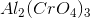.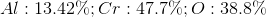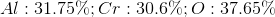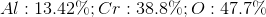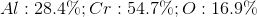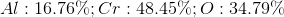Explanation:

The total mass of one mole of aluminum (II) chromate is calculated by: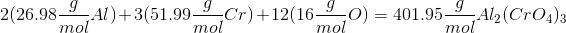Aluminum, chromate, and oxygen contribute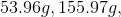and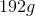respectively. Therefore, we can divide each contribution by the total molecular mass to determine percentages by mass.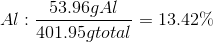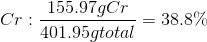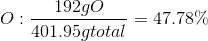### Example Question #6 : Ap Chemistry

What is the percent by mass of hydrogen in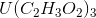?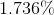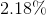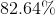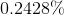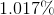Explanation:

The total mass ofis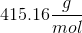and calculated by: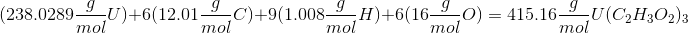You can then divide hydrogen's contribution by the total molecular mass in order to find the percent mass.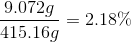### Example Question #7 : Ap Chemistry

What percent of the overall mass is represented by the cation(s) in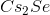?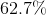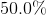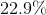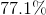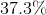Explanation:

The total molar mass ofis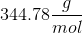. Cesium has a +1 charge when the compound is dissolved in solution and is, thus, the cation.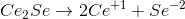Cesium contributes 265.8g to the total molecular mass.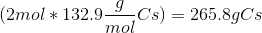We can find the percent by mass of cesium by dividing the mass of cesium by the total molecular mass.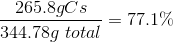### Example Question #1 : Intermolecular Forces

List the following bond types in order of increasing strength: nonpolar covalent bonds, ionic bonds, hydrogen bonds, polar covalent bonds

hydrogen bonds, nonpolar covalent bonds, polar covalent bonds, ionic bonds

nonpolar covalent bonds, polar covalent bonds, hydrogen bonds, ionic bonds

hydrogen bonds, ionic bonds, polar covalent bonds, nonpolar covalent bonds

ionic bond, polar covalent bonds, nonpolar covalent bonds, hydrogen bonds

hydrogen bonds, ionic bonds, nonpolar covalent bonds, polar covalent bonds

hydrogen bonds, ionic bonds, polar covalent bonds, nonpolar covalent bonds

Explanation:

A hydrogen bond is not a proper chemical bond, but the result of dipole-dipole interactions. While they are very chemically important, hydrogen bonds are dynamic, rather than stagnant. This is the least stable type of bond listed.

Covalent bonds are inherently more stable than ionic bonds as electrons are shared between both bound atoms, so the next stronges bond type is the ionic bond.

Chemists distinguish between covalent and ionic bonds for the sake of simplicity, but there is actually a continuum. Polar covalent bonds are on the continuum between pure ionic bonds and pure covalent bonds, so polar covalent bonds have more ionic character than nonpolar covalent bonds, and thus are less stable than nonpolar covalent bonds.

### Example Question #2 : Intermolecular Forces

Which of the following compounds experiences the greatest intermolecular forces

Benzyl Alcohol

Glucose

Ethyl Alcohol

Ammonia

Formaldehyde

Ammonia

Explanation:

IMF strength is in the order of ion-ion>h-bond>dipole-dipole>van der waals. Of the listed compounds there aren't any that display ion-ion IMF, and only ammonia has h-bonding, making it the one with the strongest forces.

### Example Question #1 : Intermolecular Forces

Order the following compounds from lowest boiling point to highest:

He2 (helium gas)

Isobutyl alcohol

Acetone

Water

Isobutyl alcohol, water, acetone, helium gas

Helium gas, acetone, water, isobutyl alcohol

Acetone, helium gas, water, isobutyl alcohol

Acetone, helium gas, isobutyl alcohol, water